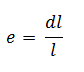# Difference Between Stress and Strain in Strength of Materials

In mechanics of solids, the knowledge of difference between stress and strain is very important. When an external force is applied on a body, it starts to deform. the external force applied on the body induces a
resisting force within the body, when this resisting force is taken on unit area is called stress. There is a change in the dimension of the body due to the induced stress. The ratio of this change in dimension with the original dimension of the body is called strain.

### Difference Between Stress and Strain in Tabular FormS.no Stress Strain 1. Stress is defined as the resisting force per unit area. Strain is defined as the deformation per unit area. 2. The ratio of resisting force ( or applied load) to the cross section area of the body is called stress. The ratio of change in dimension of the body to the original dimension is called strain. 3. Stress is denoted by the Greek symbol ‘σ’ ( sigma). Strain is denoted by the symbol ‘e’. 4. The formula of stress is given byThe formula of strain is given by5. The unit of stress is N/m2 or N/mm2. The strain is a unitless quantity. 6. Stress can exist without strain. Strain cannot exist without stress. 7. The various types of stress are: tensile stress, compressive stress and shear stress. The various types of strain are: tensile strain, compressive strain, shear strain and volumetric strain.

### Comparison Between Stress and Strain

• Stress is the resisting force per unit area where as strain is the deformation per unit area.
• The stress is given by the ratio of resisting force to the cross section area of the body. The strain is given by the ratio of change in dimension to the original dimension.
• The stress is denoted by the symbol ‘σ’ and strain by ‘e’.
• The unit of stress is N/m2 or N/mm2 whereas the strain does not have units.
• The stress can exist without strain but the existence of strain without stress in not possible.

This is all about the difference between stress and strain in strength of materials. If you find any suggestion regarding this article then you can comment us. If you found this article informative or useful than don’t forget to share and like.